# Texas Go Math Grade 2 Lesson 11.2 Answer Key One Dollar

Refer to our Texas Go Math Grade 2 Answer Key Pdf to score good marks in the exams. Test yourself by practicing the problems from Texas Go Math Grade 2 Lesson 11.2 Answer Key One Dollar.

## Texas Go Math Grade 2 Lesson 11.2 Answer Key One Dollar

Explore

Draw the coins. Write the total value.

FOR THE TEACHER • In the first box, have children draw eight nickels and then count to find the total value. In the second box, have children draw eight dimes and then count to find the total value.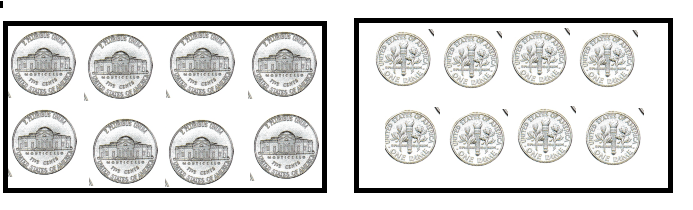Explanation:
1 Nickel = 5 cents
5 x 8 = 40 cents
1 dime = 10 cents
8 x 10 = 80 cents

Math Talk
Mathematical Processes

How many pennies have the same value 80¢? Explain.
80 pennies have the same value for 80 cents
1 penny = 1 cent

Model and Draw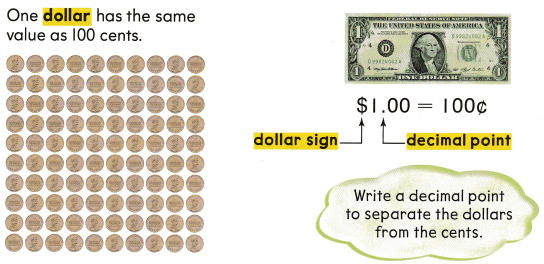Share and Show

Draw the coins to show $1.00. Write the total value.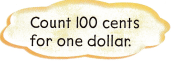Question 1. nickels Answer: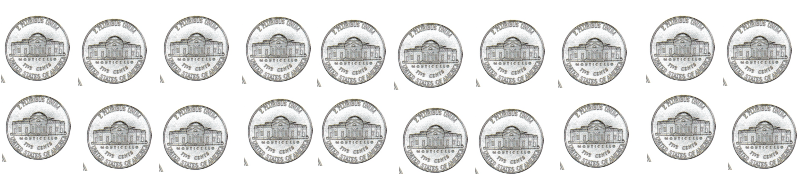Explanation: 1 nickel = 5 cents 5 x 20 = 100Cents Question 2. quarters Answer: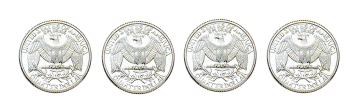Explanation: 1 quarter = 25 cents 4 x 25 = 100 cents Question 3. dimes Answer:Explanation: 1 dime = 10 cents 10 x 10 = 100 cents Problem Solving Circle coins to make$1.00. Cross out the coins you do not use.

Question 4.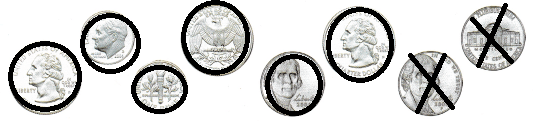Explanation
1 quarter = 25 cents
1 dime = 10 cents
1 nickel = 5 cents
3 x 25 = 75
2 x 10 = 20
1 x 5 = 5
75 + 20 + 5 = 100

Question 5.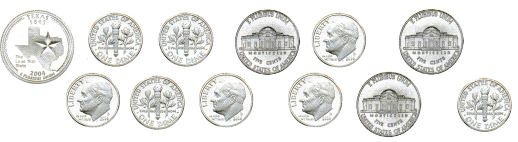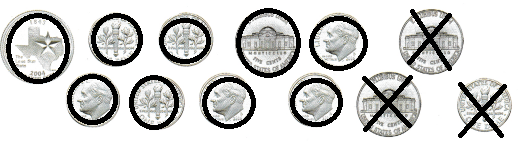Explanation:
1 quarter = 25 cents
1 dime = 10 cents
1 nickel = 5 cents
1 x 25 = 25
1 x 5 = 5
7 x 10 = 70
70 + 25 + 5 = 100

Question 6.
H.O.T. Sara has these coins. Draw more coins to show $1.00.Answer: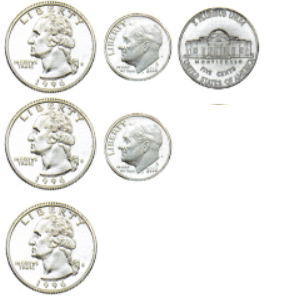Explanation: 1 quarter = 25 cents 1 dime = 10 cents 1 nickel = 5 cents 3 x 25 = 75 2 x 10 = 20 1 x 5 = 5 75 + 20 + 5 = 100 Cents Question 7. H.O.T. Multi-Step Warren shows$1.00 using only two kinds of coins. Draw and 1 label coins he could use.Explanation:
1 quarter = 25 cents
1 dime = 10 cents
1 nickel = 5 cents
25 x 3 = 75
5 x 5 = 25
75 + 25 = 100

Question 8.
Representations Victor writes one dollar as 100c. Which is another way to write one dollar?
(A) $100 (B)$1.00
(C) $1¢ Answer: B Explanation:$1.00 is another way to write one dollar

Question 9.
Multi-Step Gwen has 8 climes. How many more dimes does she need to have one dollar?
(A) 2 more dimes
(B) 1 more dime
(C) 10 more dimes
Explanation:
1 dime = 10 cents
10 x 10 = 100 cents

Question 10.
TEXAS Test Prep Mary has these coins. Which coin does she need to show $1.00?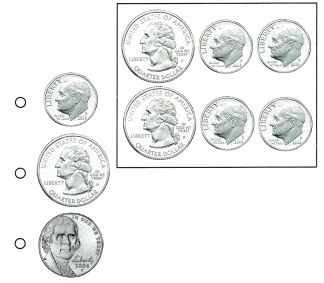Answer: A Explanation: 1 dime more is used 25 x 2 = 50 4 x 10 = 40 100 – 90 = 10 so 1 dime is used. TAKE HOME ACTIVITY • Have your child draw a group of coins to show$1.00.